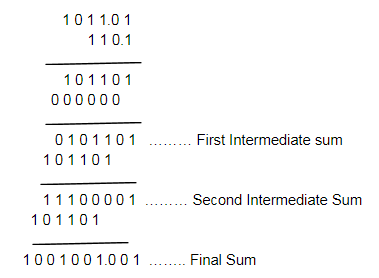# Binary Multiplication

Binary multiplication is one of the four binary arithmetic. The other three fundamental operations are addition, subtraction and division. In the case of a binary operation, we deal with only two digits, i.e. 0 and 1. The operation performed while finding the binary product is similar to the conventional multiplication method. The four major steps in binary digit multiplication are:

 0 × 0 = 0 0 × 1 = 0 1 × 0 = 0 1 × 1 = 1

Note: The binary product of the two binary numbers 1 and 1 is equal to 1 only. And no additional number is borrowed or carried forward in this operation.

Based on the above specification, we will solve here the problems online based on the multiplication of binary numbers.

In this section, you will get answers for the questions about binary multiplication, including:

• What is Binary multiplication?
• Procedure to multiply two binary numbers
• Steps to Solve Binary multiplication using examples

## What is Binary Multiplication?

The binary multiplication operation is actually a process of addition and shifting operation. This process has to be continued until all the multiplier is done, and finally, the addition operation is made.

Similar to the decimal system, the multiplication of the binary numbers is done by multiplying the multiplicand with the multiplier. It is noted that the multiplication by zero makes all the bits zero, and this step may be ignored in the intermediate steps. The multiplication by 1 makes all the multiplicand value unchanged.

### Binary Multiplication Table

The multiplication table for binary numbers is given below.

 Binary Number Multiplication Value 0 x 0 0 1 x 0 0 0 x 1 0 1 x 1 1

## Binary Multiplication Rules

Binary multiplication, like other binary operations, is much easier, unlike the decimal multiplication when you remember the following multiplication rules. The rules of binary multiplication are:

• 0 × 0 = 0
• 0 × 1 = 0
• 1 × 0 = 0
• 1 × 1 = 1 [No borrow or carry method is applicable here]

As per these rules, it very clear, that if the binary multiplication includes 0, then it will result in zero itself. Hence,

Binary product of 0 and 0 is equal to 0

Binary product of 0 and 1 is equal to 0

Binary product of 1 and 0 is equal to 0

But,

Binary product of 1 and 1 is equal to 1.

To differentiate the rules for multiplication of binary numbers from other binary operations such as addition, subtraction and division, please refer to below given table:

 Addition Subtraction Division 0+0 = 0 0-0 = 0 0÷0 = 0 0+1 = 1 0-1 = 1 (1 is borrowed) 0÷1 = 0 1+0 = 1 1-0 = 1 1÷1 = 1 1+1 = 0 (1 is carry forwarded) 1-1 = 0

## Examples of Binary Multiplication

Some binary multiplication examples are given below for a better understanding of this concept.

Example 1: Solve 1010 × 101

Solution:

1010 × 101

1010

(×) 101

—————–

1010

0000

——————

01010 ……. First Intermediate Sum

1010

——————–

110010

Comparison with Decimal values:

10102 = 1010

10102 = 510

10 x 5 = 5010

(110010)2 = 5010

Another example of binary multiplication with a decimal point is as follows:

Question: 1011.01 × 110.1

Solution:Here, the decimal point is placed three places from the least significant bit. Because, the binary number 1011.01, the decimal point is 2 places from the LSB and 110.1 the decimal point is placed 1 place from the LSB.

### Binary Multiplication Questions

Solve the below-given questions using multiplication rules, based for binary numbers.

1. Multiply 10001 x 111
2. Solve 10101 x 110
3. Find the value of 11111 x 10000

For more information on binary operations like addition, subtraction, and division operations register with BYJU’S -The Learning App and also watch exciting videos to learn with ease.

Q1

### What is Binary Multiplication?

Binary multiplication, unlike decimal subtraction, involves only two digits, i.e. 0 and 1. Visit BYJU’S to learn everything about binary multiplication.

Q2

### What are the Rules of Binary Multiplication?

There are four rules of binary multiplication which are:

• 0 × 0 = 0
• 0 × 1 = 0
• 1 × 0 = 0
• 1 × 1 = 1
Q3

### How to do binary multiplication?

To solve multiplication problems based on binary numbers, we have to use the four primary rules designated for this operation. For example, if we have to multiply 110 by 100, then we get;
110
X     100
——–
000
000
110
———-
11000
———
Q4

### What are the steps for binary multiplication?

The binary multiplication is very much similar to the usual multiplication method of integers. First, we need to multiply each digit of one binary number to each digit of another binary number. And then add them all together to get the final result.
Q5

### What is the binary product of 1001 and 1011?

The binary product of 1001 and 1011 is 1100011.
Quiz on Binary Multiplication